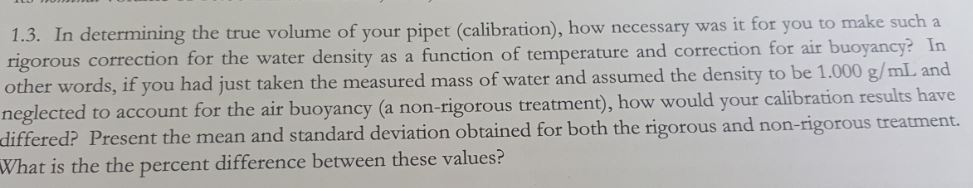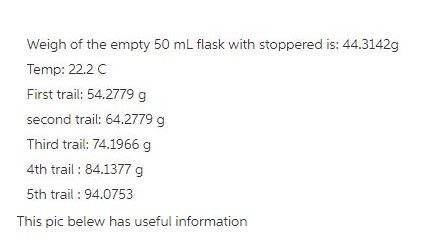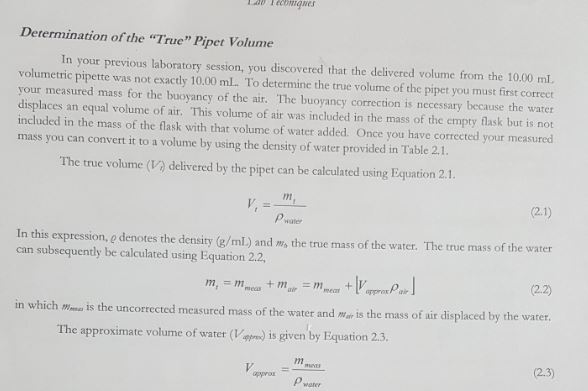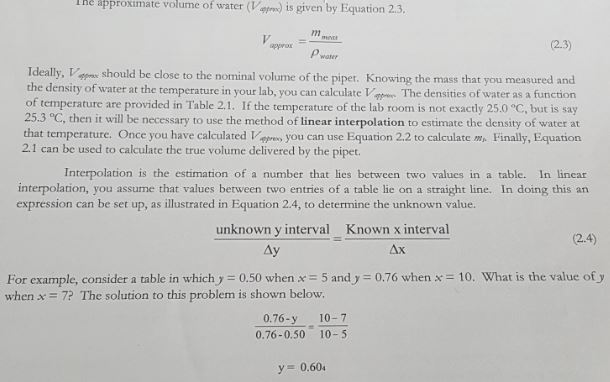# Question & Answer: 1.3. In determining the true volume of your pipet (calibration), how necessary was it for you to…..Don't use plagiarized sources. Get Your Custom Essay on
Question & Answer: 1.3. In determining the true volume of your pipet (calibration), how necessary was it for you to…..
GET AN ESSAY WRITTEN FOR YOU FROM AS LOW AS \$13/PAGE1.3. In determining the true volume of your pipet (calibration), how necessary was it for you to other words, if you had just taken the measured mass of water and assumed the density to differed? Present the mean and standard deviation obtained for both the rigorous and non-rigorous treatment. What is the the percent difference between these values? for the water density as a function of temperature and correction for air buoyancy? In t for the air buoyancy (a non-rigorous treatmen), how would your calibration results have

In determining the true volume of pipet, it is necessary to make a rigorous correction for the water density as a function of temperature in order to achieve the accurate readings.

Rigorous treatment

The density of water at 22.2 oC = 0.997724 g/mL

The given measurements can be converted to volumes by subtracting the empty flask weight (i.e. 44.3142) and dividing by density of water (i.e. 0.997724 g/mL)

1. (54.2779 – 44.3142)/0.997724 = 9.9864 mL

2. (64.2779 – 44.3142)/0.997724 = 20.0092 mL

3. (74.1966 – 44.3142)/0.997724 = 29.9506 mL

4. (84.1377 – 44.3142)/0.997724 = 39.9143 mL

5. (94.0753 – 44.3142)/0.997724 = 49.8746 mL

The mean of given measurements (x̄) can be calculated as shown below.

x̄ = (9.9864 + 20.0092 + 29.9506 + 39.9143 + 49.8746)/5, i.e. 29.9470

The sample standard deviation for the above measurements (s) can be calculated as shown below.

s = {(9.9864 – 29.9470)2 + (20.0092 – 29.9470)2+ (29.9506 – 29.9470)2 + (39.9143 – 29.9470)2 + (49.8746 – 29.9470)2) / (5-1)}1/2, i.e. 15.7610

Non-rigorous treatment

The given measurements can be converted to volumes by subtracting the empty flask weight (i.e. 44.3142) and dividing by density of water (i.e. 1 g/mL)

1. (54.2779 – 44.3142)/1 = 9.9637

2. (64.2779 – 44.3142)/1 = 19.9637

3. (74.1966 – 44.3142)/1 = 29.8824

4. (84.1377 – 44.3142)/1 = 39.8235

5. (94.0753 – 44.3142)/1 = 49.7611

The mean of given measurements (x̄) can be calculated as shown below.

x̄ = (9.9637 + 19.9637 + 29.8824 + 39.8235 + 49.7611)/5, i.e. 29.8789

The sample standard deviation for the above measurements (s) can be calculated as shown below.

s = {(9.9637 – 29.8789)2 + (19.9637 – 29.8789)2+ (29.8824 – 29.8789)2 + (39.8235 – 29.8789)2 + (49.7611 – 29.8789)2) / (5-1)}1/2, i.e. 15.7252

The percent difference between the mean values obtained by rigorous and nonrigorous treatments

= {(29.9470​ – 29.8789)/29.9470}*100, i.e. ​0.2274%

The percent difference between the standard deviation values obtained by rigorous and nonrigorous treatments

= {(15.7610​ – 15.7252)/15.7610}*100, i.e. ​0.2271%